# Frequency Selection in Switching Power Supply Designs (Part I)Get valuable resources straight to your inbox - sent out once per month

## Introduction

Frequency — a basic property of switching power supplies — represents the rate at which the DC voltage switches on and off. Understanding switching frequency is foundational to the working principles of power lines in practical applications. This article is the first part of a two-part series delving into switching frequency design.

Part I will discuss calculating for the key variables of switching frequency, as well as the challenges with higher frequencies. Part II will cover how to design a switching power supply for frequency ranges in practical applications.

## Principles Related to Switching Frequency

Switching power supplies use a switching action to transform DC power into a specific frequency of pulsed current energy. The electrical energy is released according to predetermined requirements, and the inductive and capacitive energy stored in components. Similar to how our pulses can indicate our health, both regular and self-regulating switching frequencies indicate the quality of the switching power supply (see Figure 1). Therefore, switching frequency is one of the critical indicators for a switching power supply.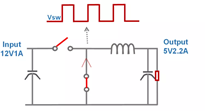Figure 1: Switching Frequency as an Indicator of Switching Power Supply Quality

The regular switching action is the primary mechanism during switching power supply operation, and the frequency plays a decisive role in the circuit calculations. Consider a buck circuit where the frequency (fS) determines the inductor current ripple (ΔIL) and output voltage ripple (VRIPPLE). fS and the ripple amplitude are approximately inverse, meaning a higher frequency corresponds with a smaller ripple. ΔIl can be calculated using Equation (1):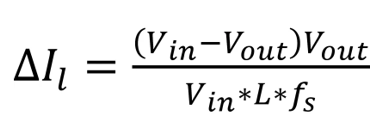VRIPPLE can be estimated using Equation (2):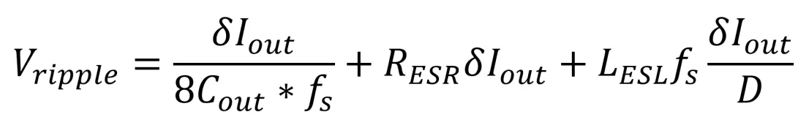Equation (2) shows that fS, the inductance (L), and the product of fS and the output capacitor (COUT) are all used as calculation factors.

The stored inductance and capacitance energies can be calculated using Equation (3):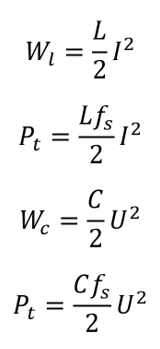Under the same conditions, increasing fS can simultaneously reduce the inductance and capacitance while optimizing the device’s volume. Increasing fS means less energy can be stored at a time, which reduces the requirements for energy storage components. In addition, a higher fS reduces VRIPPLE while improving power quality.

## Challenges of Increasing Frequency

Increasing fS also increases losses, and directly affects three core indicators: switching power supply loss, efficiency, and heat. Consider a buck circuit where the majority of loss consists of the on loss, switching loss, and driving loss. In particular, the switching frequency directly affects switching loss and driving loss.

Driving loss is the drive current that is lost by the MOSFET’s parasitic capacitors (CGS and CGD) under the drive voltage (see Figure 2). This loss mostly comes from the MOSFET tube’s parasitic charge and discharge (QG).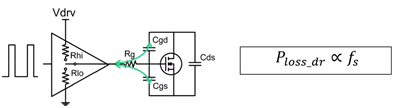Figure 2: Loss of Drive Current

The driving loss can be estimated with Equation (4):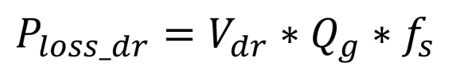For switching loss, take the MOSFET tube’s opening process as an example. Each time the MOSFET turns on, the voltage and current change. Figure 3 shows that the switching loss is proportional to the switching frequency, where the overlapping area between VDS and IDS indicates the switching loss.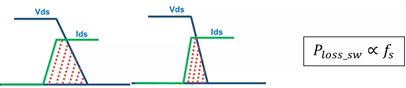Figure 3: Switching Loss between Voltage and Current Changes

Designers must reduce the switching loss to improve the switch’s opening and closing edge speeds, increase how quickly IDS and VDS change, and directly reduce the overlapping area. Switching loss can be estimated using Equation (5):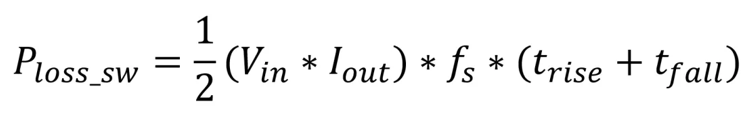However, this method aggravates the high-frequency square wave, leading to an adverse impact on high-frequency EMI. The Fourier transform of the switch’s quasi-square wave signal demonstrates that fS, as well as the switch’s rising and falling edge speeds, significantly influence the signal gain. As fS increases, so does the system’s harmonic distortion, which leads to EMI (see Figure 4). To improve efficiency at higher frequencies, EMI design must be strictly controlled.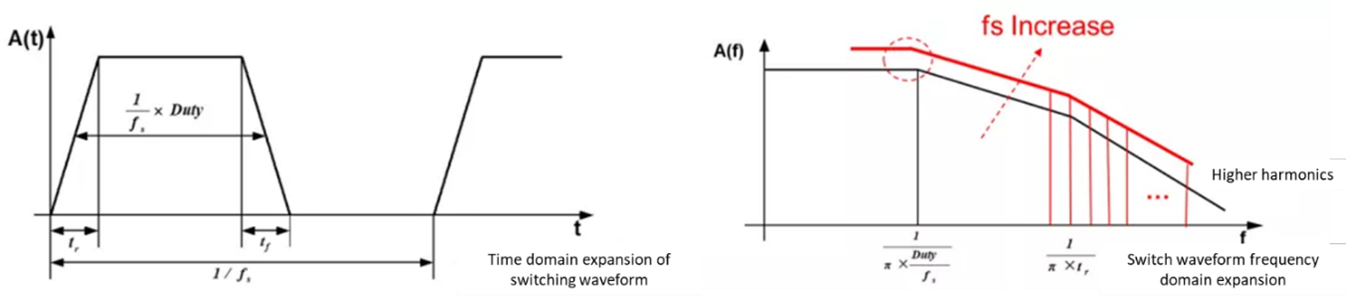Figure 4: Increasing Frequency Leads to EMI Deterioration

In the circuit EMI design, the fixed-frequency switching power supply has a single frequency point, so the energy is concentrated near the frequency point and the doubled frequency point, resulting in higher EMI noise. To optimize EMI, frequency jitter can be used within a specific range to disperse the noise signal’s energy, which reduces noise peaks (see Figure 5).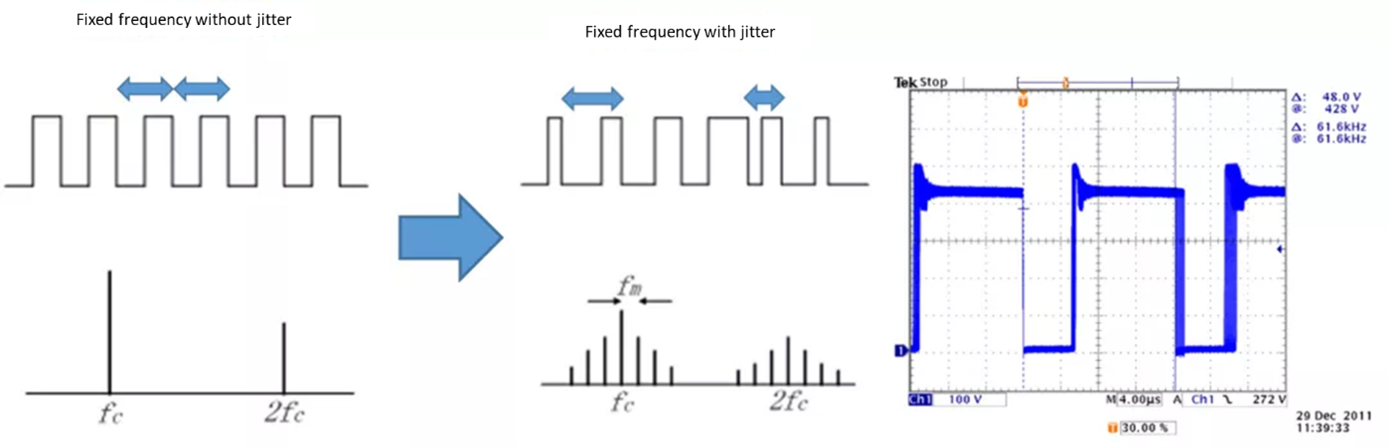Figure 5: Optimize EMI with Frequency Jitter

As EMI solutions evolve, high-frequency designs become more complex. In addition to the frequency jitter design, designing a light-load switching frequency for a power supply requires careful consideration. For example, the differences between fixed-frequency mode and adjustable frequency mode are reflected in the circuit's dynamic characteristics, light-load efficiency, and static power consumption. Based on the actual application scenarios, these differences should be determined at the beginning of the power supply design process.

## Conclusion

In this article, we covered approaches to calculate the core circuit indicators that are influenced by the switching frequency. We also addressed the different types of loss that result from higher frequencies. Part II will discuss practical application scenarios with three different frequency ranges. By understanding the fundamentals of the switching power supply frequency design, power engineers can improve the power quality and the efficiency of energy storage components.

### Did you find this interesting? Get valuable resources straight to your inbox - sent out once per month!

Get technical support